# An Introduction to Static Scheduling for MIMD Architectures

Henry G. Dietz, Matthew T. O'Keefe, and Abderrazek Zaafrani

School of Electrical Engineering
Purdue University
West Lafayette, IN 47907
hankd@ecn.purdue.edu

## Abstract

Recently, we have developed a technique whereby a new class of MIMD architectures called "Barrier MIMDs" can use static scheduling to remove over 77% of all synchronizations from traditional MIMD code [ZaD90] . The new static scheduling technique combines a VLIW-like code scheduling [Ell85] technique with new relative timing analysis that allows the compiler to determine when synchronization constraints can be met without introducing runtime synchronization barriers. However, the use of the new barrier MIMD hardware is critical to this technique, since it provides a mechanism for maintaining and/or regaining precise timing information whereas conventional MIMD computers merely provide directed synchronization.

In this paper, we review the techniques for Barrier MIMD and discuss the application of the same method to reducing synchronization in conventional, directed-synchronization, MIMD computers. This includes discussions of NOP insertion and timing analysis for directed synchronization. A scheduling algorithm and preliminary experimental results are also presented. ( This work was supported in part under National Science Foundation grant no. 8624385-CDR. )

Keywords: Static Barrier MIMD (SBM), Dynamic Barrier MIMD (DBM), barrier synchronization, code scheduling, compiler optimization.

## Notice for HTML Users

The HTML version of this paper differs from the standard postscript version (click here for the postscript version) in several ways, including a variety of minor formatting changes. The standard postscript version was formatted by ```groff -mgs``` as a single file. In contrast, this HTML document was generated using a script that replaces figures with in-line `gif` images that are hypertext linked to full-resolution postscript versions; the `(`n`K .ps.Z)` at the upper right of each in-line image provides both the size of the compressed postscript file and the hypertext link. There are also a variety of hypertext links inserted for navigation within the document, including a Hypertext Index at the end of this document (click here to jump to the Hypertext Index).

## 1. Introduction

Runtime synchronization overhead is a critical factor in achieving high speedup using parallel computers. A key advantage of SIMD (Single Instruction stream, Multiple Data stream) architectures is that synchronization is effected statically at compile-time, hence the execution-time cost of synchronization between "processes" is essentially zero. VLIW (Very Long Instruction Word) [Ell85] , [CoN88] machines are successful in large part because they preserve this property while providing more flexibility in terms of the operations that can be parallelized. Unfortunately, VLIWs cannot tolerate any asynchrony in their operation; hence, they are incapable of parallel execution of multiple flow- paths, subprogram calls, and variable-execution-time instructions. In a recent paper [DiS89] , a new architecture, Barrier MIMD, was proposed to extend the static synchronization properties of the SIMD and VLIW class of parallel machines into the MIMD domain.

A Barrier MIMD is a MIMD computer which has specialized hardware implementing a new type of barrier synchronization which aids the compiler in performing static scheduling. If a barrier is placed across a set of processes, then no process can execute past that barrier until all have reached the barrier. Unlike other barrier mechanisms, all processes will resume execution in exact synchrony. Hence, immediately after executing a barrier, the machine can be treated as a VLIW, using static scheduling to eliminate the need for further runtime synchronization.

However, VLIW machines do not allow MIMD code structures (e.g., multiple flow paths) nor even variable-time instructions. In a barrier MIMD machine, static scheduling tracks both minimum and maximum completion times for each processes' code; runtime synchronization is needed iff the minimum time for the consumer of an object is less than the maximum time for that object's producer. If the timing constraints cannot be met statically, this implies that the static timing information has become too "fuzzy." Inserting another barrier effectively reduces this fuzziness to zero. Scheduling and barrier placement algorithms for barrier MIMDs have been developed and extensively benchmarked; more than 77% of all synchronizations in the benchmark programs were accomplished without runtime synchronization in a barrier MIMD (see figure 16).

The goal of this paper is to show how the timing analysis and scheduling concepts which were designed for Barrier MIMD machines can be applied to more conventional, directed synchronization, MIMD architectures. While conventional MIMD machines clearly cannot achieve the full benefit obtained by statically scheduling for Barrier MIMD computers, similar timing analysis and scheduling can be applied to achieve a significant reduction in conventional MIMD synchronization.

This work summarizes a code scheduling algorithm for barrier MIMDs, an "optimal" barrier insertion algorithm, extensive scheduling experiments on synthetic benchmarks using the new algorithms, and how these techniques can be applied to conventional MIMD machines.

The structure of the paper is that section 2 introduces the basic concepts of timing analysis and static code scheduling for various types of MIMD computers. Detailed timing analysis and code scheduling algorithms are given for Barrier MIMD machines in section 3; performance determined by thousands of scheduling experiments is discussed in section 4. Section 5 discusses performance differences between different architectures, especially between Barrier MIMD and VLIW architectures. Because Barrier MIMD are the idealized target for such techniques, the work in sections 3, 4, and 5 provides an excellent overview of the general technique as well as bounds on its performance for other MIMD architectures. Finally, section 6 summarizes the contributions of this work and the direction of current research efforts.

## 2. Timing Analysis for MIMD

As suggested above, the primary new contribution in the current paper is the concept of applying Barrier MIMD analysis and scheduling techniques to more conventional directed-synchronization MIMD computers. The differences in hardware structure correspond to significant differences in the structure of the timing problems. In this section, we briefly review the basic principles behind using timing information in scheduling code for a Barrier MIMD and then give a detailed analysis of the timing information available in directed synchronization MIMDs.

### 2.1. Synchronization vs. Timing

The traditional view of synchronization is that each consumer of a datum must wait until after the producer of that datum has executed. In other words, synchronization is viewed as enforcing an order of execution. Figure 1 gives a multiprocess time line showing the use of conventional directed synchronization to insure that the consumer executes after the producer. The directed arc from the producer to the consumer represents the runtime transmission of a synchronization object from the producer to the consumer. Process 0 simply generates this message and continues execution, whereas process 1 cannot proceed past the head of the arc until the message from the producer has arrived. This transmission could take a potentially unbounded amount of time dependent on, for example, routing and traffic through a network; hence, in the traditional view, the only timing information available at compile-time is that the consumer will execute at some time after the producer.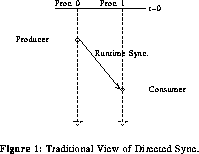( 4K .ps.Z )

However, this traditional view ignores the fact that some timing information is available to the compiler. If compiler analysis attempts to precisely track the minimum and maximum times at which the producer and consumer would execute relative to time t=0, then runtime synchronization may be unnecessary. As shown in figure 2, if the minimum consumer time is greater than the maximum producer time, then no runtime synchronization is required.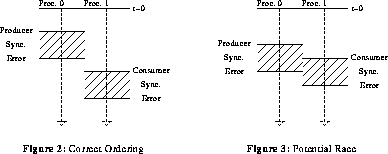( 5K .ps.Z )

However, as shown in 2.2. NOP Insertion

Perhaps the most obvious alternative is to change the code schedule so that some additional instructions are inserted just before the consumer, much as in pipeline scheduling [NiD90] [Gro83] . However, a good code scheduler (such as that presented in section 3) would have taken this into consideration in generating the original schedule, hence there are no additional useful instructions available for use as padding. Instead, one would simply insert enough NOPs (Null OPerations) before the consumer so that the minimum consumer time is greater than the maximum producer time. Sufficient NOPs must be inserted to yield an execution time of at least max(producer) - min(consumer). This is depicted in figure 4.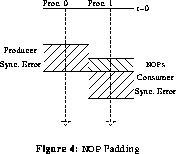( 4K .ps.Z )

However, there are two unavoidable disadvantages to using NOP insertion to meet timing constraints:

1. The NOPs are executed, and delay introduced, even when the particular run does not require the delay. For example, in 2.3. Barrier Insertion

In essence, a Barrier MIMD scheduler also inserts delays to achieve timing constraints, but the delays (barriers) are structured so that:

1. Each barrier introduces delay only when needed and only for as long as needed to satisfy the timing constraint in that run; there is no unnecessary delay.
2. Each barrier eliminates (or at least reduces to a fixed bound) the range between minimum and maximum time in each process participating in the barrier.

Figure 5 shows the primary effect of inserting a barrier to enforce the timing constraint.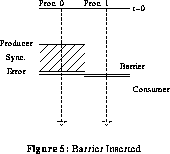( 4K .ps.Z )

However, inserting a barrier has an important side-effect. Insertion of a barrier not only enforces a timing constraint, but also reduces the difference between minimum and maximum times for the participating processes. This results in an effect unique to the combination of barriers and our timing analysis: inserting one barrier may cause several apparently unrelated timing constraints to be resolved. Consider the producers and consumers of figure 6. When a barrier is inserted to enforce the first timing relationship, the second is also resolved because the narrowing of the range between minimum and maximum times for the second producer and consumer allows the compiler to determine that they are properly ordered.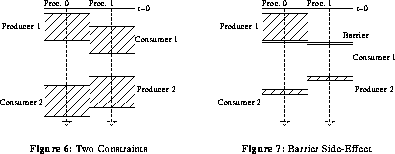( 6K .ps.Z )

According to the studies presented in section 4, this effect satisfies approximately 28% of all timing constraints.

The primary disadvantage in using Barrier MIMD scheduling is that it only applies directly to machines which have hardware implementing this new type of barrier -- currently, only the PASM prototype [ScN87] . No doubt, other machines will eventually be built to incorporate this mechanism, e.g., the CARP machine [DiS89a] ; still, the problem of applying the timing analysis and scheduling to existing MIMD machines remains.

### 2.4. Directed Synchronization

As discussed in section 2.1, the only synchronization mechanism in many MIMD computers is a directed synchronization primitive which enforces an after timing relationship.

Shaffer [Sha89] and others have applied a transitive reduction [AhH74] to task graphs to remove redundant directed synchronization in code executing on MIMD architectures. Callahan [Cal87] proposed a similar method for reducing the number of (conventional) barrier synchronizations required in scheduling nested loop constructs. The timing analysis which we propose not only recognizes these redundancies (see Figure 8), but also redundancies based purely on timing, without a subsuming synchronization.( 4K .ps.Z )

In terms of timing, if process 0 sends a message to process 1, and process 1 waits for the message to arrive before proceeding, this enforces the timing constraint that the portion of process 1 which occurs after receipt of the message from process 0 also happens after the portion of process 0 which was executed before sending the message. Notice that the synchronization is not symmetric relative to processes 0 and 1; the synchronization is directed from process 0 to process 1.

Figure 9 clarifies this timing relationship. The figure shows that:

• Process 0 executes for between a and b units of time before sending the directed synchronization to process 1
• Process 1 executes for between c and d units of time before it begins waiting for the synchronization message from process 0
• The synchronization message must travel from process 0 to process 1, hence, transmission delay time may vary between e and f (due to network traffic, etc.)

Because the process which sends the directed synchronization does not wait for the receiving process, ( In some MIMD computers the process sending a directed synchronization message waits for an acknowledgement from the receiving process. This is modeled as a pair of directed synchronizations, and is discussed in section 2.5 under the title "Simulated Barriers." ) the time at which the first instruction after the synchronization is executed in process 0 is simply (a, b) -- there is no delay introduced. However, process 1 might find the message waiting for it or might have to wait for the message to arrive; hence, the time when the first instruction after the synchronization is executed by process 1 is (max(a+e, c), max(b+f, d)).( 4K .ps.Z )

This yields some interesting properties:

1. Clearly, unlike using a synchronization barrier, timing for the sending process (process 0) is unaffected by the directed synchronization.
2. The minimum to maximum time range for the receiving process (process 1) can be reduced for some, but not all, values of a, b, c, d, e, and f.

This second point is significant in that it suggests that conventional MIMD architectures might be able to obtain benefits similar to those obtained using timing analysis with the new Barrier MIMD architecture.

There is, however, a fundamental problem with the use of our timing analysis and directed synchronization. Suppose that the consumer process executes a while loop, with unbounded execution time, before it consumes the value from the producer. Relative to figure 9, this has the effect of making d=infinity, ( Here, we use infinity to represent an unboundedly large particular number, not literally infinity. ) but this is turn makes the time for the first consumer process instruction after the synchronization be (max(a+e, c), infinity). Notice, however, that the situation is not symmetric.

If the producer process executes an unbounded while loop before sending the synchronization message, then b=infinity. Assuming that d<infinity, the fact that b=infinity implies that for the worst-case time, the critical path to the point where the synchronization message is consumed will be the path of length b+f: the consumer process will have to wait for the synchronization message to arrive. If the critical path always includes the message transmission from the producer, i.e., a+e>=d, then the actual values for a and b are immaterial. In other words, if a+e>=d then we know that, immediately after the synchronization, the consumer process is at time (e,f) relative to the producer process (with no further analysis needed).

First, consider the timing assignments shown in figure 10. A preliminary examination of the timing suggests that the producer occurs at time (5+3, 7+4) = (8,11) and the consumer occurs at time (max(5+1, 7), max(7+3, 8)) + 4 = (11,14). Since these times overlap, it appears that an additional synchronization is needed to enforce the ordering, however, the critical path analysis mentioned above proves that the timing is safe. The producer process region before the synchronization is on the critical path in the overlap timing; recognizing this fact, it is easily seen that the producer occurring at time 11 implies that the consumer occurs in the time interval (12,14), hence no timing overlap is possible and no additional synchronization is needed.

Figure 11 demonstrates that this principle can be used to preserve static timing constraints even in the presence of unbounded-time operations such as the afore-mentioned while loop. The only difference between figures 10 and 11 is that the producer process region which is on the consumer's critical path has an unbounded maximum time, (5,infinity). This changes nothing, however, because if the maximum time for the producer is infinity+4, the minimum time for the consumer is infinity+1+4, one time unit later. Hence, no additional synchronization is needed. In fact, we can completely ignore the infinity, instead saying that the producer executes at time (3,4) and the consumer executes between time max(5+1, 7) - 5 + 4 = 6 and time max(infinity+3, 8) - infinity + 4 = 7. Since (3,4) and (6,7) do not overlap, no additional synchronization is needed.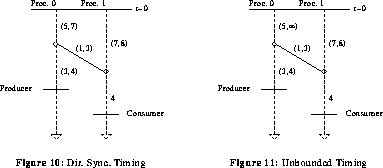( 5K .ps.Z )

This analysis is vital to using timing in most existing MIMD computers, because most of these machines have processors which must occasionally service interrupts and other unpredictable, variable-delay, events. By enabling this event handling only on a critical path just before a directed synchronization message is sent, even infinitely- long delays can be accepted without losing accuracy or precision in static timing analysis.

### 2.5. Simulated Barriers

Another alternative for existing MIMD computers is to use directed synchronization to simulate the new barrier mechanism. Unfortunately, the simulated barriers have much higher overhead than the Barrier MIMD hardware barriers, and this limits the use of simulated barriers for fine-grain code.

A binary (two-process) barrier can be simulated using two directed synchronizations, as shown in figure 12. In effect, the same critical path timing analysis discussed in the previous section is employed to insure that both the producer and consumer code regions before the simulated barrier are on the final critical path, hence, unbounded execution times in either can be "absorbed." Notice that in order for this to occur, it is necessary that h<=e+i, since violating this timing bound could remove the consumer region from being on the critical path for the producer.

Of course, the simulated barrier differs significantly from a Barrier MIMD hardware barrier in that it is not symmetric, has significantly higher overhead, and does not provide exact timing (unless directed synchronization takes a fixed amount of time). In effect, the simulated barrier acts as shown in figure 13.( 6K .ps.Z )

In figure 13, the barrier is conceptually initiated by process 0 up to e+i units of time before synchronization is desired, likewise, process 1 initiates its barrier i units of time before synchronization is achieved. Although this pre-issuing is somewhat awkward to implement, the primary effect is that of creating a barrier which has a timing error of j-i.

## 3. Code Scheduling and Timing Analysis Algorithms

In this section, we describe code scheduling and timing analysis algorithms for Dynamic Barrier MIMDs (DBMs). The appropriate modification for the static barrier MIMD (SBM) is to merge unordered barriers with execution time overlap. Adaptation of these techniques for NOP insertion and directed synchronization is significantly more complex, hence, only a cursory discussion is given in this paper.

Although minimum and maximum times are known for each of the instructions instead of a fixed execution time, it is straightforward to adapt the scheduling heuristics commonly used for fixed-execution-time tasks -- and this has been our approach. It is well-known that even for simple or relaxed cases the optimal static scheduling of a partially ordered set of tasks on parallel processors is NP-hard, and hence, computationally intractable [KaN84] . However, several heuristics with bounded worst-case performance degradation (from optimal) have been found to be effective for this problem [Hu82] . In particular, the critical path method exhibits good performance at reasonable computational cost.

Some useful terminology is given in section 3.1. Section 3.2 outlines a technique for labeling operations and section 3.3 applies these labels to generate an ordering for list scheduling. Using the list ordering, section 3.4 details the assignment of operations to specific processors. Upon assigning each operation to a processor, it may be necessary to insert a barrier; algorithms for this purpose are given in section 3.5.

### 3.1. Terminology

Several terms that will be used later in this paper are now defined.

Total Implied Synchronizations:
The number of edges in the directed acyclic graph (DAG) corresponding to the code generated from a basic block. Each edge is considered to be a producer/consumer synchronization pair.
Barrier Synchronization Fraction:
The number of barriers in the schedule divided by the Total Implied Synchronizations.
Serialized Synchronization Fraction:
The number of synchronizations satisfied by serialization, i.e., a consumer assigned to the same processor as a producer, divided by the Total Implied Synchronizations.
Static Scheduling Fraction:
The remaining fraction of total implied synchronizations after the barrier and serialized fractions are removed. This represents the synchronizations that are scheduled away by tracking static timing constraints after a barrier executes, as in the second producer/consumer synchronization of figure 7 .

The partial ordering among barriers can be modeled as a directed acyclic graph. This model is developed in separate papers [OKD90a] , [OKD90b] that appear in these proceedings; these papers also describe barrier MIMD hardware design. The two types of barrier MIMD mentioned in this paper, static barrier MIMD (SBM) [OKD90a] and dynamic barrier MIMD (DBM) [OKD90b] , differ in that the SBM imposes a total order on barrier execution at run-time, while the DBM does not constrain the run-time execution order of the barriers.

### 3.2. Node Labeling

The scheduling algorithm assumes that the instructions are represented in an instruction DAG G(N, A), where N is the set of n instruction nodes and A is the set of edges representing the precedence (producer/consumer) constraints between instructions. If an edge is directed from node i to node j, node j is said to be a successor (or consumer) of node i. Similarly, node i is said to be a predecessor (or producer) of node j.

The DAG is assumed to have one entry and one exit node; dummy exit or entry nodes (with zero execution time) are added as necessary to satisfy this condition. Let t(i) represent the execution time for node (instruction) i, which is assumed to take integral values. For variable-execution- time instructions, tmin(i) and tmax(i) represent the minimum and maximum execution times, respectively, for node i. For the instruction DAG, the critical path is defined as the longest path from the entry node to the exit node, expressed mathematically as( 3K .ps.Z )

where k represents the kth path from the entry node to the exit node. Clearly, tcr represents a lower bound on the execution time of the instruction DAG, regardless of the number of processors that execute it. The height of node i is defined as the length of the longest path from the exit node to node i where the orientation, or direction, of the edges are reversed, i.e.,( 4K .ps.Z )

where k represents the kth path from the exit node to node i.

For the variable-execution-time instructions in the DAG, the minimum and maximum height for a node i, hmin(i) and hmax(i), are defined as follows:( 4K .ps.Z )

The minimum height corresponds to the height for node i assuming all nodes in the DAG take their minimum execution time and similarly, the maximum height corresponds to the height for node i assuming all nodes in the DAG take their maximum execution time.

### 3.3. Node Ordering

The maximum height and minimum height are computed for all nodes. This can be done in O(n2) time, since the problem reduces to finding longest paths from the exit node to all other nodes [Hu82] (with edge orientations reversed). The nodes are first sorted into a list in nonincreasing order using the maximum height as the key, and ties between nodes with equal maximum height are broken using the minimum height (largest first) as the key. The complexity of this sort procedure is no worse than O(nlog2n) [AhH74] .

### 3.4. Node Assignment

During this phase, the nodes are removed (in order) from the sorted list and assigned to particular processors. Some nodes should be placed in a processor that includes a predecessor (producer) for that node. This serialization of the nodes increases efficiency because it reduces the number of processors required and may eliminate a run-time synchronization operation. On the other hand, too much serialization can increase the schedule length. The node assignment algorithm attempts to strike a balance between these two competing aims.

In the following description of the node assignment and barrier insertion algorithms, the current node being scheduled is referred to as node i. The processor to which some node j is assigned is denoted as Processor(j).

1. The first step in node assignment is to determine the set of processors in which the predecessors of node i, denoted as Preds(i), are scheduled. These are referred to as the producer processors for i, or ProdProc(i). Go to step .
2. For each processor in ProdProc(i), determine if no other nodes are scheduled after Pred(i) on that processor. If no processor meets this condition, go to step . If only a single producer processor meets this condition, place node i in that processor, and insert a barrier if necessary, as described in the next section. If more than one producer processor meets this condition, assign node i to the producer processor with the largest current maximum time (to possibly avoid inserting a barrier). If all processors in ProdProc(i) have the same current maximum time, choose one at random and assign i to it.
3. Assign node i to a processor such that it is scheduled as early as possible. In case of ties between processors, choose one at random. This helps balance the number of nodes assigned to each processor. Insert a barrier as necessary.

### 3.5. Barrier Insertion

Two algorithms for barrier insertion are described. The first algorithm is conservative in that it always adds a barrier synchronization when one is necessary, but it may add unnecessary, redundant barriers. The other barrier insertion algorithm is "optimal" in the sense that a barrier is not inserted unless it is absolutely necessary at the time of the insertion. The barrier dag (B,<b) [OKD90a] is constructed incrementally as the nodes are assigned to processors and barriers inserted into the schedule.

The notion of one barrier "dominating" another is useful in constructing the barrier dag [AhS86] . A barrier x dominates barrier y, written x dom y, if every path from the initial node of the barrier dag to y goes through x. With this definition, the initial barrier dominates all other barriers in the dag and every barrier dominates itself.

Each edge (u,v) between barriers u and v in a barrier dag contains the minimum and maximum execution time for the code between the barriers.

After consumer node i has been assigned to a processor C, it is necessary to check all producers for i to determine if a barrier is necessary. Suppose that node g is a member of the set Preds(i), the predecessors, or producers, for instruction i, and that it is assigned to processor P.

#### 3.5.1. Conservative Barrier Insertion

To determine if a barrier is needed between instruction nodes i and g, assigned to processors C and P, respectively, the following steps are performed:

1. Define LastBar(g) as the last barrier to execute before node g. Define NextBar(g) to be the next barrier to execute after node g. Check for a path between NextBar(g) and LastBar(i). Let us call this procedure PathFind(g,i). ( This step is similar to the removal of transitive synchronization edges between the producer and consumer employed by Shaffer [Sha89] and others. However, our technique combines scheduling with the transitive reduction step, so that unnecessary transitive edges are never added to the barrier graph. ) If a path is found, no barrier is needed; otherwise, continue with step .
2. Find the nearest common dominating barrier for barriers LastBar(g) and LastBar(i), written as CommonDom(g,i). Go to step .
3. The length of the longest path, assuming maximum execution times for code regions between barriers, from CommonDom(g,i) to LastBar(g) is computed. Denote this longest path as max(CommonDom(g,i),LastBar(g)), and its length as l(max(CommonDom(g,i),LastBar(g))). Add the maximum time necessary to execute all instructions after LastBar(g) up to and including g, denoted as max(g), to this length to yield the maximum time Tmax(g) to execute node g relative to the common dominating barrier. Go to step .
4. Similarly, the longest path, assuming minimum execution times for code regions, from the common dominator to LastBar(i) is computed. Denote this longest path as min(CommonDom(g,i),LastBar(i)), and its length as l(min(CommonDom(g,i),LastBar(i))). Let i- represent the instruction before i on processor C. The minimum time to execute all instructions up to but not including i, denoted as min(i-), is added to this length, yielding the minimum time Tmin(i-) to start executing node i relative to the common dominator. Go to step .
5. If Tmin(i-)>=Tmax(g), then no barrier is needed; otherwise, go to step .
6. A barrier is inserted across processor P somewhere after the producer node g, and across processor C just before node i. To determine where the barrier is placed on P, we compute l(max(CommonDom(g,i),LastBar(i))), and then add in the maximum execution times of all instructions on C after LastBar(i) up to node i-, yielding Tmax(i-). If Tmax(i-)<=Tmax(g), then the barrier is inserted right after the producer node g on processor P. Node i is scheduled right after the barrier just inserted. However, if Tmax(i-)>Tmax(g), and if there is some instruction g+ after instruction g, such that Tmax(i-) falls into the execution time range (assuming maximum times) of g+, then the barrier is inserted after g+ on processor P.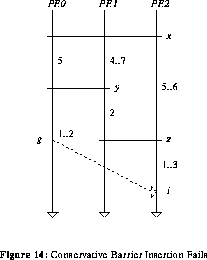( 4K .ps.Z )

This barrier insertion algorithm will sometimes add unnecessary barriers. For example, in the barrier embedding given in figure 14, the conservative insertion algorithm will insert a barrier across processors 0 and 2, after the producer node g and before consumer node i. In the figure, LastBar(g) is y and LastBar(i) is z. The common dominating barrier for y and z is x. It can be seen that Tmax(g)=9, while Tmin(i-)=8, so it would appear that a barrier is necessary.

However, the longest path from x to z, min(x,z), overlaps with the longest path from x to y, max(x,y), on edge (x,y); recall that different assumptions have been made about the execution time for this edge on the different paths. If this is taken into account, then min(x,z) should be computed, as before, assuming minimum execution times for edges except for the edges which intersect max(x,y). For these edges, the maximum execution time should be used when computing the longest path. In figure 14, this means that edge (x,y) has value 7, (y,z) has value 2, and the minimum time for i- is 1, yielding an actual minimum time for node i of 10. Thus, i always executes after g and no barrier is required. In the next section, an optimal barrier insertion algorithm that does not generate these unnecessary barriers is described.

#### 3.5.2. Optimal Barrier Insertion

From the previous example, it is clear that the problem with the conservative insertion algorithm is that it does not take into account the possibility that the longest paths from the common dominator to the producer and consumer nodes may overlap. In such cases, assuming maximum execution times on edges that overlap may increase the minimum execution time for the consumer node just enough to resolve the synchronization statically. (This problem is closely related to the critical path issues discussed in section 2.4 relative to timing analysis for directed synchronization operations.)

Let u be the nearest common dominator for barriers v and w, where v is LastBar(g) and w is LastBar(i). Recall that node i is being scheduled, node g is a producer for node i, and it is necessary to determine if a barrier is required between these instructions.

The relationship between the various path lengths can be expressed as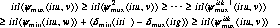( 4K .ps.Z )

where max(u,v),2<=j<=k represents the jth longest path (assuming maximum execution times) from u to v. For each max(u,v), find min(u,w), the longest path from u to w assuming minimum execution times except for edges on the path max(u,v), where maximum execution time edges are used. If the condition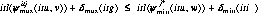( 4K .ps.Z )

is satisfied, consider the next longest path max(u,v) and repeat the process. If the condition is not met, then a barrier must be inserted as described in step  of the conservative barrier insertion algorithm, and the scheduling algorithm starts again with the next node in the list.

This process of successively checking the jth longest paths continues until( 4K .ps.Z )

is met for the kth longest path, proving that the synchronization is satisfied statically and no barrier is required.

## 4. Scheduling Experiments

The scheduling algorithms discussed in the last section were applied to the synthetic benchmark programs. The effects of varying different parameters that are related to the architecture of the machine and the structure of the synthetic benchmarks have been studied. Architecture parameters that were varied include the number of processors and timing assigned to each instruction; barriers were assumed to always execute immediately upon arrival of the last participating processor. Benchmark parameters included the number of instructions and variables in generated programs. Particular attention has been paid to the different synchronization fractions and how they vary as the parameters change. These results have provided good feedback concerning the performance of the scheduling algorithms.

### 4.1. Structure of the Synthetic Benchmark Programs

This study focuses on fine-grain scheduling of a single-chip multiprocessor RISC node [DiS89a] that employs the barrier mechanism discussed in this paper. Expensive operations such as multiplication and division are implemented as data- dependent code sequences that introduce asynchrony into the chip operation. Memory accesses across a shared bus or interconnection network involve contention that also involves stochastic delays. It is shown that static scheduling may still be used to advantage within this framework.

In this work, we wished to characterize and study the extent to which static scheduling can be employed in barrier MIMDs. In particular, measurements of the number of synchronizations that are satisfied statically, at compile- time, versus the number that require explicit synchronization instructions executed at run-time were desired. To this end, a compiler was developed for a simple language consisting of basic blocks of code with no control flow constructs.

The programs to be scheduled on barrier machines were automatically generated using common instruction execution frequencies [AlW75] . This allowed us to automatically generate a very large number of synthetic benchmarks from which summary statistics were obtained. It also made it quite simple to change the various characteristics of generated programs to observe the effects on the statistics of scheduled programs. The drawback, of course, is that it is not possible to take real benchmark programs directly as input. Current efforts include prototype compiler development to generate barrier MIMD code for standard programming language constructs.

#### 4.1.1. Benchmark Instruction Set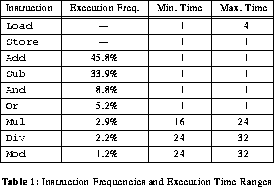( 4K .ps.Z )

The scheduling algorithm takes as input a basic block of instructions. A basic block is a region of code that contains a sequence of consecutive statements. This region should have a single entry point and no embedded control structures [AhS86] . There are nine instructions generated from the synthetic code sequences in the instruction set: four of these nine instructions have variable execution time. For the other operations, it is realistic to assume that they have a constant execution time of one unit. These operations are Or, And, Add, Sub, and Store. Table 1 summarizes the instruction frequencies and execution time ranges.( 4K .ps.Z )

#### 4.1.2. Benchmark Synthesis

A C program was developed to randomly generate the basic blocks according to the statistics mentioned previously. This program requires as input the number of statements, variables, and constants desired in the generated code. It then generates a random sequence of assignment statements satisfying the desired conditions. The frequency of the assignment statements corresponds loosely to the instruction frequency distributions found in [AlW75] . Note that in table 1 the frequencies of load and store are not given. These instructions are provided as necessary during code generation and optimization: the first reference to a variable causes a load for that variable to be generated, and a store is generated when a variable is assigned a value.( 5K .ps.Z )

During code generation, the randomly-generated assignment statements are optimized using standard local optimizations, including common subexpression elimination, constant folding and value propagation, and dead code elimination [AhS86] . Hence, the resulting synthetic benchmark does not contain "redundant" parallelism that might skew the results.

An example synthetic benchmark is shown in table 2, and its corresponding DAG (Directed Acyclic Graph) is shown in figure 15 . In table 2, the leftmost column represents the tuple number. Each tuple is incrementally assigned a number as it is produced by the code generator. Many tuples are not represented because they were removed by the optimizer. The two rightmost columns represent the minimum finish time and maximum finish time assuming unlimited parallelism. These columns will help in ordering the tuples as will be explained in section 4. In the instruction DAG, the instructions are represented as nodes while edges represent the precedence constraints between instructions. This DAG is important to both code optimization and the scheduling algorithm.

### 4.2. Experimental Results

One-hundred synthetic benchmarks were generated for each set of parameters and the results averaged to yield each point on the curves shown in this section. Over the course of the study, thousands of benchmarks were generated. Over all these benchmark programs, the results fell into the following ranges:

* The barrier fraction varies from 3% to 23%.
* The serialization fraction varies from 50% to 90%.
* The fraction of barriers statically scheduled away varies from 8% to 40%.

Note that the last fraction represents a feature unique to Barrier MIMD architectures.

While the NOP insertion and directed synchronization timing- based code scheduling techniques will achieve some fraction of static scheduling removing barriers, as an approximation to the barrier mechanism, they are bounded by the Barrier MIMD performance. In other words, all of the performance data can be directly applied to general MIMD architectures by simply treating the serialization as the minimum performance and the Barrier MIMD performance (serialization + static scheduling) as the maximum performance. This leaves a relatively wide bound in many cases, however, this is not surprising in that it reflects the fact that there are many additional issues for directed synchronization machines; for example, performance will depend strongly on the inherent grain size of the code being larger than the typical variation in synchronization message transmission time. Hence, we seek to find bounds on performance for all types of MIMD machines by investigating in detail the performance of Barrier MIMD machines.

Perhaps the best summary of the Barrier MIMD scheduling results is a scatter plot with the serialization fraction on the vertical axis and the statically scheduled fraction on the horizontal axis, as shown in figure 16. The results for more than 2000 of the synthetic benchmarks (each benchmark contained between 65 and 132 synchronizations) are given in the figure, and it can be seen that the "center of mass" of the points lies near the 85% line; hence, on average about 85% of the synchronizations for the benchmarks in the plot are either serialized or statically scheduled away.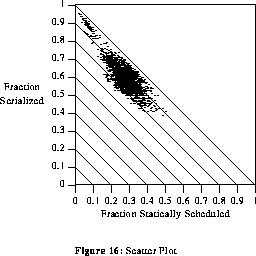( 17K .ps.Z )

#### 4.2.1. Number of Assignment Statements

In this section, the number of processors and variables are fixed (8 processors and 15 variables), while the number of instructions varies. This section investigates the effect of changing the number of instructions in the synthetic benchmarks.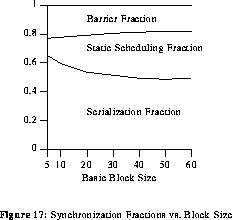( 4K .ps.Z )

As can be seen in figure 17, the fraction of barrier synchronization decreases as the number of statements in the generated basic blocks increases. This decrease is relatively large when the number of statements varies from 5 to 20. Generally speaking, the Load operations are scheduled for early execution. Since Load is a variable- execution-time instruction (from 1 and 4 time units), barriers are often required after a Load. Hence, there is a concentration of barriers at the the beginning of the execution of the basic block. The barrier fraction decreases when we increase the number of statements because the percentage of Loads becomes smaller. This effect is counter-balanced at larger benchmark sizes because Mul, Div, and Mod instructions begin appearing in generated benchmarks, and these instructions have large execution time variation.

Notice that the serialization fraction decreases as the benchmark size increases. Larger benchmarks make it less likely that a consumer can be placed directly after a producer because another instruction has probably been scheduled there already.

#### 4.2.2. Number of Variables

In this section, the benchmark size and number of processors are fixed (60 statements and 8 processors), while number of variables is changed. Referring to figure 15, as the number of variables increases, the barrier fraction first increases, then remains constant. Since the parallelism width is within a constant factor (0.65) of the number of variables, the parallelism width increases with the number of variables. Hence, the scheduling algorithm employs more processors in the schedule as the number of variables increases. The result is that more barriers are generated until the parallelism width of the benchmark exceeds the number of processors, and the barrier fraction then almost becomes constant.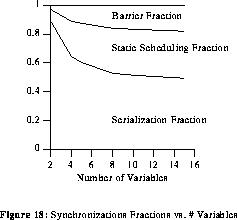( 4K .ps.Z )

The serialization fraction decreases when more variables are used because for a large number of variables, the parallelism width is large, and fewer opportunities for serialization exist.

#### 4.2.3. Number of Processors

In this section, the benchmark size and number of variables are fixed, while the number of processors is varied. For two variables, increasing the number of processors did not affect the barrier fraction because the scheduling algorithm keeps almost all of the instructions in two processors. The serialization and static scheduling fractions also remain constant.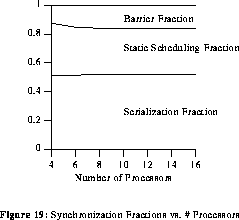( 4K .ps.Z )

For five variables, when the number of processors is increased from two to four the barrier fraction increases because more synchronization is required between the different processors. The barrier fraction stabilizes after four processors as the scheduling algorithm employs no more than four processors. For N variables, the barrier fraction increases when the number of processors employed is less than the parallel width. When the number of processors used is greater than the parallelism width, the barrier fraction remains constant.

Figure 19 illustrates the effect of increasing the number of processors on the different synchronization fractions for a barrier machine. This figure is for 100-assignment statement basic blocks with 10 variables. As can be seen in the figure, the serialization fraction remained nearly constant as the number of processors increased. There are two effects contributing to this serialization rate behavior that tend to cancel each other out, resulting in a fixed serialization fraction. The first effect is that for small numbers of processors, the scheduling algorithm often inadvertently serializes a synchronization operation. Conversely, the scheduling algorithm has fewer opportunities to serialize for a barrier machine with a small number of processors, because quite often the "serialization slot" will already be filled. The first effect results in an decrease in the serialization fraction for larger numbers of processors while the second effect yields an increase in the serialization fraction.

## 5. Architecture Performance Comparisons

Since we have suggested extension of static scheduling from VLIW machines to various types of MIMD architectures, it is useful to examine the relative performance. In this section, we present the results of an experimental comparison between Barrier MIMD and VLIW execution, as well as discussing the performance differences expected for more conventional MIMD architectures.

The experimental evaluation of VLIW versus Barrier MIMD performance was made using the same code scheduling techniques and synthetic benchmarks used in section 4. Figure 20 shows the results.( 5K .ps.Z )

In the VLIW execution mode all instructions were assumed to require their maximum time to execute. No asynchrony was allowed in VLIW execution. As can be seen in the figure, the maximum times for both the barrier MIMD and VLIW were nearly identical. Execution time as displayed in figure 20 has been normalized to VLIW execution time. The barrier machine took slightly longer to complete execution for smaller numbers of processors because more barriers were required due to instruction timing variation.

The minimum barrier MIMD completion time was about 25% lower than the VLIW completion time, and average barrier completion time will fall between the minimum and maximum times, the exact value being determined by the probability distributions of the variable-execution-time instructions. It should be noted that an optimal schedule (completion time equal to the critical path time) was determined for almost all the synthetic benchmarks for the comparison.

These results suggest that it is important to reduce the maximum execution time for instructions, and this is traditionally done in VLIWs by adding extra hardware for such operations as integer and floating-point multiplies. Also, memory reference delays are reduced by having multiple paths to memory banks and through careful scheduling of memory references to avoid bank conflicts [CoN88] .

In considering how more traditional (directed synchronization) MIMD computers will perform, the basic issue is one of grain size. The large timing variations in some MIMD directed synchronization message transmission times make fine-grain parallel code synchronization intensive (or heavily serialized or laden with NOPs). In such cases, the execution time might become significantly longer than for a comparable VLIW; however, if grain size can be made large enough so that the variations in message transmission times are negligible, a directed synchronization MIMD should perform very much like a DBM. We are currently investigating these effects.

## 6. Conclusions

VLIW architectures have proven to be capable of providing consistently good performance over a larger range of programs than vector processors. However, VLIW architectures cannot achieve efficient parallel execution of while loops, subroutine calls, and variable-execution-time instructions. The new Barrier MIMD hardware, in concert with new relative timing analysis and code scheduling techniques, has shown great potential in reducing synchronization cost while still supporting these code structures, eliminating over 77% of all synchronizations (see figure 16), but current MIMD machines do not support Barrier MIMD operation [OKD90a] [OKD90b] .

In this paper, we have introduced the basic concepts involved in using the new timing analysis and code scheduling for a more general class of MIMD architectures. Detailed algorithms and experimental performance evaluation of the techniques for Barrier MIMD machines are given, further, it is suggested how these techniques can be adapted and approximate performance predicted for more general MIMD architectures.

There is however, far more work to be done. Ongoing work includes the extension of the basic scheduling techniques to more complex code structures (including arbitrary control flow), development of efficient timing analysis and code scheduling techniques for various types of MIMD computers, and construction of prototype compilers for use in experimental evaluation of performance. We are also working toward a complete machine design, the CARP (Compiler- oriented Architecture Research at Purdue) machine [DiS89a] , that incorporates the Barrier MIMD mechanism as well as various other novel compiler/architecture interactions.

## References

[AhS86]
A. V. Aho, R. Sethi, and J. D. Ullman, Compilers: Principles, Techniques, and Tools, Addison-Wesley, Reading, MA, 1986.
[AhH74]
A.V. Aho, J.E. Hopcroft, and J.D. Ullman, The Design and Analysis of Computer Algorithms. Addison-Wesley, Reading, MA, 1974.
[AlW75]
W.G. Alexander and D.B. Wortman, "Static and Dynamic Characteristics of XPL Programs," IEEE Computer, November 1975, pp. 41-46.
[Cal87]
C.D. Callahan II, A Global Approach to the Detection of Parallelism, Ph.D. Dissertation, Rice University, March 1987.
[CoN88]
R. P. Colwell, R. P. Nix, J. J. O'Donnell, D. B. Papworth, and P. K. Rodman, "A VLIW Architecture for a Trace Scheduling Compiler," IEEE Trans. on Computers, vol. C-37, no. 8, pp. 967-979, Aug. 1988.
[DiS89]
H. G. Dietz, T. Schwederski, M. T. O'Keefe, and A. Zaafrani, ""Extending Static Synchronization Beyond VLIW," Supercomputing 89, pp. 416-425, Reno, NV, Nov. 1989.
[DiS89a]
H.G. Dietz, H.J. Siegel, W.E. Cohen, M.T. O'Keefe, et al., "A Compiler-Oriented Architecture: The CARP Machine," Fourth SIAM Conference on Parallel Processing for Scientific Computing, Chicago, IL, December 1989.
[Ell85]
J. R. Ellis, Bulldog: A Compiler for VLIW Architectures. Cambridge, MA: MIT Press, 1985.
[Gro83]
T. Gross, "Code Optimization Techniques for Pipelined Architectures," COMPCON '83, Spring 1983.
[Hu82]
[KaN84]
H. Kasahara and S. Narita, "Practical Multiprocessor Scheduling Algorithms for Efficient Parallel Processing," IEEE Trans. on Computers, vol. C-33, no. 11, pp. 1023-1029, November 1984.
[NiD90]
A. Nisar and H. G. Dietz, "Optimal Code Scheduling for Multiple-Pipeline Processors" 1990 International Conference on Parallel Processing, August 1990.
[OKD90a]
M. T. O'Keefe and H. G. Dietz, "Hardware Barrier Synchronization: Static Barrier MIMD (SBM)" 1990 International Conference on Parallel Processing, August 1990.
[OKD90b]
M. T. O'Keefe and H. G. Dietz, "Hardware Barrier Synchronization: Dynamic Barrier MIMD (DBM)" 1990 International Conference on Parallel Processing, August 1990.
[ScN87]
T. Schwederski, W. G. Nation, H. J. Siegel, and D. G. Meyer, "The Implementation of the PASM Prototype Control Hierarchy," Proceedings of the Second International Conference on Supercomputing, vol. I, 1987, pp. 418-427.
[Sha89]
P.L. Shaffer, "Minimization of Interprocessor Synchronization in Multiprocessors with Shared and Private Memory," Proc. 1989 Int. Conf. Parallel Processing, vol. III, pp. 138-142, Aug. 1989, St. Charles, IL.
A. Zaafrani, H. Dietz, and M. O'Keefe, "Static Scheduling for Barrier MIMD Architectures," 1990 International Conference on Parallel Processing, St. Charles, IL, August 1990.

## Hypertext Index

Abstract
Notice for HTML Users
1. Introduction
2. Timing Analysis for MIMD
2.1. Synchronization vs. Timing
2.2. NOP Insertion
2.3. Barrier Insertion
2.4. Directed Synchronization
2.5. Simulated Barriers
3. Code Scheduling and Timing Analysis Algorithms
3.1. Terminology
3.2. Node Labeling
3.3. Node Ordering
3.4. Node Assignment
3.5. Barrier Insertion
3.5.1. Conservative Barrier Insertion
3.5.2. Optimal Barrier Insertion
4. Scheduling Experiments
4.1. Structure of the Synthetic Benchmark Programs
4.1.1. Benchmark Instruction Set
4.1.2. Benchmark Synthesis
4.2. Experimental Results
4.2.1. Number of Assignment Statements
4.2.2. Number of Variables
4.2.3. Number of Processors
5. Architecture Performance Comparisons
6. Conclusions
References
Hypertext Index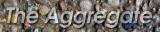The only thing set in stone is our name.